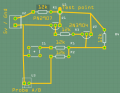# Need help calculating maximum current in circuit

#### parhallenberg

Joined Dec 19, 2015
10
Hi!

I'm making a modified version of the water leak detector from Make #28, please see attached image for my circuit. Right now it's working, with a LED (between Test point and GND) lighting up on when detecting water or when activating test switch. Now I want to calculate the maximum current that could rush through the circuit, before I dare to connect Test point to an input pin on the Arduino UNO (I would also like to be able to supply 5V from an output pin, which also depends on maximum current draw).

Since I'm new to PNP transistors, I'm not even sure where to start. Which route is the important one for me? Is it A) 5V --> PN2907 --> Test point --> R5 --> GND? (And in that case, maybe I should put a resistor between 5V and PN2907 Collector to minimize current to Test point?) Or is it B) 5V --> Test switch --> R1 --> R4 --> Test point --> R5 --> GND?
A)
I = V / R5
V = 5V
R5 = 12k
I = 0,42mA

B)
I = V / (R1 + R4+ R5)
V = 5V
R1 + R4 + R5 = 36k
I = 0,14mA#### bertus

Joined Apr 5, 2008
22,142
Hello,

This way we can see what you are doing.

Bertus

#### parhallenberg

Joined Dec 19, 2015
10
Hello,
Bertus
Sorry, yes of course. Not sure if decent, though. Usually I do them on paper. This is from Fritzing:
U1 is my test point. U2 is GND and 5V. U3 is two test probes, which will act like a closed switch if put in water. S1 is test switch (NO). And Q1 is PN2907, Q2 is 2n3904.
View attachment 96842

#### Alec_t

Joined Sep 17, 2013
13,436
When Q1 switches on, tens of mA could flow into test point U1 if it is a low-impedance input of the Arduino. The current is determined by the gain (beta) of Q1.